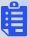Question 1

1/-0

If F = 7, H = 343, J = 16807 then I + G = _______.

SOLUTION

 7 ? 343 ? 1607 F G H I J 71 = 3 72 = 49 73 = 343 74 = 2401 75 = 16807

I + G = 49 + 2401 = 2450

Question 2

1/-0

A shaving set company sells 4 different type of razors. Elegance, Smooth, Soft and Exceutive. Elegance sells at Rs. 48, Smooth at Rs. 63, Soft at Rs. 78 and Executive at Rs. 173 per piece. The table below shows the numbers of each razor sold in each quarter of a year.

 Quarter/Product Elegance Smooth Soft Executive Q1 27300 20009 17602 9999 Q2 25222 19392 18445 8942 Q3 28976 22429 19544 10234 Q4 21012 18229 16595 10109

Which product contributes the greatest fraction to the revenue of the company in that year?

SOLUTION

 Quarter/Product Elegance Smooth Soft Executive Q1 27300 20009 17602 9999 Q2 25222 19392 18445 8942 Q3 28976 22429 19544 10234 Q4 21012 18229 16595 10109 Total Sale 102510 80059 72186 39284 Total revenue 4920480 5043717 5630508 6796132

From the revenue numbers it is clear that the maximum revenue is by sale of executive type razor.

Question 3

1/-0

If a prime number on division by 4 gives a remainder of 1, then that number can be expressed as

SOLUTION

A prime number on division by 4 gives a remainder of 1

Let the prime number is x.

Now, we can express the number as

x = 4n + 1

Let us consider the prime numbers that satisfies the above equation i.e. x = 5, 13, 17, 29, 37, 41, 53, …

x = 5 = 12 + 22

x = 13 = 22 + 32

x = 17 = 12 + 42

x = 29 = 22 + 52

x = 37 = 12 + 62

x = 41 = 42 + 52

x = 53 = 22 + 72Therefore, x can be expressed as the sum of squares of two natural numbers.

Question 4

1/-0

In a school, 60% of students play cricket. A student who does not play cricket, plays football. Every football player has got a two-wheeler. Which of the following conclusions cannot be drawn from the above data?

1. 60% of the students do not have two-wheelers

2. No cricketer has a two-wheeler

3. Cricket players do not play football

Select the correct answer using the code given below:

SOLUTION

In a school, 60% of students play cricket.

A student who does not play cricket, plays football.

Every football player has got a two-wheeler.

1. 60% of the students do not have two-wheelers: Every football player has got a two-wheeler. It doesn’t mean that the students who play cricket don’t have two-wheelers. So, it is not definitely true.

2. No cricketer has a two-wheeler: Every football player has got a two-wheeler. It doesn’t mean that the students who play cricket don’t have two-wheelers. So, it is not definitely true.

3. Cricket players do not play football: In a school, 60 % of students play cricket. A student who does not play cricket, plays football. But there is no information about whether the students playing cricket plays football or not. So, it is not definitely true.So, we cannot draw any conclusion from the given data.

Question 5

1/-0

A traveller to the town reaches a crossroad. Upon asking residents A, B and C for directions to a certain destination, he gets the following responses.

A: turn left

B: do not turn left

C: go straight

If only one among A, B and C is truthful, the traveller

SOLUTION

Given that only one among A, B and C is truthful.

Case 1: A is truthful

A: turn left → it means we need to turn left

B: do not turn left → it means we need to turn left

C: go straight → it means we need to turn left or right

Conclusion: A is truthful, and we need to turn left

Case 2: B is truthful

A: turn left → it means we need to turn right or go straight

B: do not turn left → it means we need to turn right or go straight

C: go straight → it means we need to turn left or right

Conclusion: B is truthful, and we need to turn right

Case 3: C is truthful

A: turn left → it means we need to turn right or go straight

B: do not turn left → it means we need to turn left

C: go straight → it means we need to go straight

Conclusion: The statements are contradicting each other and hence the C is not truthful.Final conclusion: As it satisfies first two cases, he will not be able to decide between going left or right.##### Current Test Score
out ofWe are providing something unique, useful and most importantly fun. By giving students a tool to find instant solutions to their doubts, we’re trying to make every student self-sufficient in practicing & completing their homework

### Exams Study Material

NEET Study Material NEET Previous Year Papers JEE Main Syllabus×